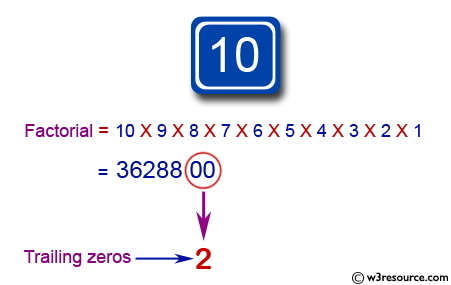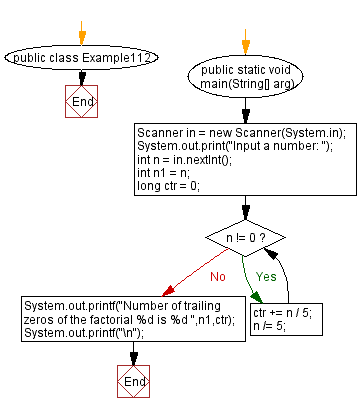﻿ Java: Compute the number of trailing zeros in a factorial# Java Exercises: Compute the number of trailing zeros in a factorial

## Java Basic: Exercise-112 with Solution

Write a Java program to compute the number of trailing zeros in a factorial.

Example
7! = 5040, therefore the output should be 1.

Pictorial Presentation:Sample Solution:

Java Code:

``````import java.util.Scanner;
public class Example112 {
public static void main(String[] arg)
{

Scanner in = new Scanner(System.in);
System.out.print("Input a number: ");
int n = in.nextInt();
int n1 = n;
long ctr = 0;
while (n != 0)
{
ctr += n / 5;
n /= 5;
}
System.out.printf("Number of trailing zeros of the factorial %d is %d ",n1,ctr);
System.out.printf("\n");
}
}
```
```

Sample Output:

```Input a number : 5040
Number of trailing zeros of the factorial 5040 is 1258
```

Flowchart:Java Code Editor:

What is the difficulty level of this exercise?

Test your Programming skills with w3resource's quiz.

﻿

## Java: Tips of the Day

countOccurrences

Counts the occurrences of a value in an array.

Use Arrays.stream().filter().count() to count total number of values that equals the specified value.

```public static long countOccurrences(int[] numbers, int value) {
return Arrays.stream(numbers)
.filter(number -> number == value)
.count();
}
```

Ref: https://bit.ly/3kCAgLb